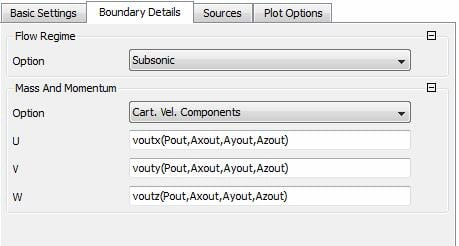## Fluids

Topics relate to Fluent, CFX, Turbogrid and more

•Min Zhang
Subscriber

There are many types of boundary conditions for outlet in CFX. However, it seems to be impossible to impose both velocity and static pressure simultaneously. Here is a problem, at the outlet B.C., the pressure and velocity are coupled:

Velocity u*Ay + miu*Velocity u. Gradient y - Ax*Ay=0               (Eq.1)

Velocity v*Ay + miu*Velocity v. Gradeint y - Ay*Ay

&               + (Velcoity•A) - 0.5*Velcoity*Velcoity = Pressure+Q  (Eq.2)

Velocity w*Ay + miu*Velocity w. Gradient y - Az*Ay=0               (Eq.3)

where A=(Ax,Ay,Az) is a predefined vector (it is defined using initialized profile data) and Q is also a given data.

Now, the outlet boundary condition needs to be defined based on Eqs.(1-3). However, the unknown variables, including the pressure and three components of the Velocity vector, are more than the available equations by one.

Before I present the question, it might be worth mentioning that during the solver run, the pressure, velocity and velocity gradient can be get using the "USER_GETVAR" routine. So the questions are:

1. since the available equations are more than the unknown variables, can I define the pressure arbitrarily and then calculate and specify the three components of the velocity at the outlet boundary condition (see the figure below, where pout=constant)?2. As pointed out by the CFX modeling guide, USER_GETVAR cannot be used to change the value of the solution field.

If the static pressure is specified at the outlet and the pressure value is calculated using Eq.(2), then after every iteration step, is there any ways to correct the solved Velocity u and Velocity w using Eq.(1) and Eq.(3) respectively? This involves to manually change the outlet velocity field.